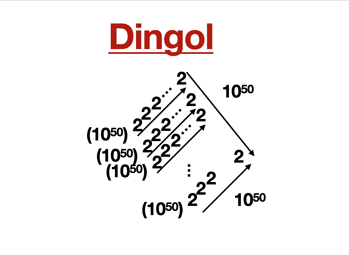11,055 PagesDingol in squares

The dingol is equal to $$r(10^{50},2)$$ in Rampant Array Notation. The number was coined by Googology Wiki user Nirvana Supermind.

## Etymology

The name is derived from googol and the prefix di- meaning 2.

## Approximations

Notation Approximation
Hyper-E notation E(2*10^50-1)#1023.4786097723456
Rampant Array Notation r(10^50,2) (exact value)
Arrow notation $$10\uparrow\uparrow(2\cdot{10^{50}}-1)$$
BEAF {10,2*10^50-1,2}
Fast-growing hierarchy $$f_3(2\cdot{10^{50}}-1)$$

## See also

Basic notation:
Community content is available under CC-BY-SA unless otherwise noted.